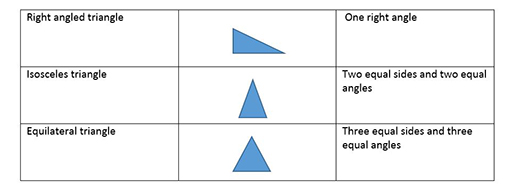Teaching mathematics

Start this free course now. Just create an account and sign in. Enrol and complete the course for a free statement of participation or digital badge if available.

Free course

# 2.1 Triangles

Triangles are the most basic of the polygons (a polygon is a straight-sided shape). They have three sides, the smallest number of sides it is possible to have. Triangles also make a rigid structure, as any engineer will know.

You will know the names of the triangles which have further properties in addition to having three sides (Figure 6).Figure 6 Types of triangle

In addition, the isosceles right-angled triangle has a right angle, two equal sides, and two equal angles of 45⁰ each.

Acute-angled triangles have three angles all smaller than a right angle.

Obtuse-angled triangles have one angle that is bigger than a right angle.

TM_1